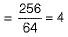Courses

# Test: File Structures- 1

## 10 Questions MCQ Test Question Bank for GATE Computer Science Engineering | Test: File Structures- 1

Description
This mock test of Test: File Structures- 1 for Computer Science Engineering (CSE) helps you for every Computer Science Engineering (CSE) entrance exam. This contains 10 Multiple Choice Questions for Computer Science Engineering (CSE) Test: File Structures- 1 (mcq) to study with solutions a complete question bank. The solved questions answers in this Test: File Structures- 1 quiz give you a good mix of easy questions and tough questions. Computer Science Engineering (CSE) students definitely take this Test: File Structures- 1 exercise for a better result in the exam. You can find other Test: File Structures- 1 extra questions, long questions & short questions for Computer Science Engineering (CSE) on EduRev as well by searching above.
QUESTION: 1

Solution:
QUESTION: 2

Solution:
QUESTION: 3

### Index sequential file is made of all of these expect

Solution:
QUESTION: 4

Which of the following is correct?

Solution:

Most database systems use indexes built on some form of a B+ tree due to its many advantages, in particular its support for range queries. Leaf nodes are linked together in B+ trees hence range queries are faster.

QUESTION: 5

B+ trees are preferred to binary trees in databases because

Solution:

Disk access is slow and B+ tree provide search in less number of disk hits. This is primarily because unlike binary search trees, B+trees have very high fanout (typically on the order of 100 or more), which reduce the number of I/O operations required to find an element in the tree.

QUESTION: 6

AB+ tree index is to be built on the Name attribute of the relation STUDENT. Assume that all student names are of length 8 bytes, disk blocks are of size 512 bytes, and index pointers are of size 4 bytes. Given this scenario, what would be the best choice of the degree (i.e. the number of pointers per node) of the B+ tree?

Solution:

Let n be the degree
Given, k, key size (length of the name = 8 byte attribute of student)
Disk block size, B = 512 bytes
Index pointer size, b = 4 bytes
Degree of B+ tree can be calculated if we know the maximum number of key a internal node can have the formula for that is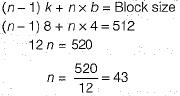QUESTION: 7

A database table T1 has 2000 records and occupies 80 disk blocks. Another table T2 has 400 records and occupies 20 disk blocks. These two tables have to be joined as per a specified join condition that needs-to be evaluated for every pair of records from these two tables. The memory buffer space available can hold exactly one block of records for T1 and one block of records for T2 simultaneously at any point in time. No index is available on either table.
Q. If Nested-loop join algorithm is employed to perform the join, with the most appropriate choice of table to be used in outer loop, the number of block accesses required for reading the data are

Solution:

Here condition given only 1 block of T1 and 1 block of T2 can present simultaneously inside the memory buffer space.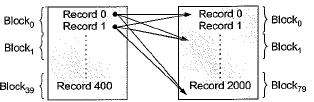In Nested loop join every record of one table every block of second table is loaded.
So, If T1 is outer = 2000 x 20 + 80 = 40080
If T2 is outer = 400 x 80 + 20 = 32020
So we go with T2 as outer table = 32020

QUESTION: 8

A database table T1 has 2000 records and occupies 80 disk blocks. Another table T2 has 400 records and occupies 20 disk blocks. These two tables have to be joined as per a specified join condition that needs-to be evaluated for every pair of records from these two tables. The memory buffer space available can hold exactly one block of records for T1 and one block of records for T2 simultaneously at any point in time. No index is available on either table.
Q. If, instead of Nested-loop join, Block nested-loop join is used, again with the most appropriate choice of table in the outer loop, the reduction in number of block accesses required for reading the data will be

Solution:

In Nested block loop join, in place of for every record of table 1, fetch every block of table 2, we go for every block of table 1, fetch every block of table 2.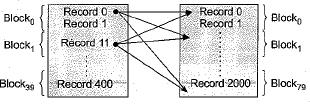So, If T1 is outer = 80 x 20 + 80 = 1680
If T2 is outer = 80 x 20 + 20 = 1620
So we go with T2 as outer table = 1620
Reduction in number of block access
= 32020-1620 = 30400

QUESTION: 9

A clustering index is defined on the fields which are of type

Solution:

If records of a file are physically ordered on a non-key field which doesn't have a distinct value for each record that field is called the clustering field.

QUESTION: 10

Consider a file of 16384 records. Each record is 32 bytes long and its key field is of size 6 bytes. The file is ordered on a non-key field, and the file organization is unspanned. The file is stored in a file system with block size 1024 bytes, and the size of a block pointer is 10 bytes. If the secondary index is built on the key field of the file, and a multilevel index scheme is used to store the secondary index, the number of first- level and second-level blocks in the multilevel index are respectively

Solution:

Total number of records = 16384
Record size = 32 B
lock size = 1024 B
Number of records inside block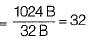In index block number of entries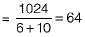So, at 1st level index block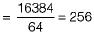at 2nd level index block